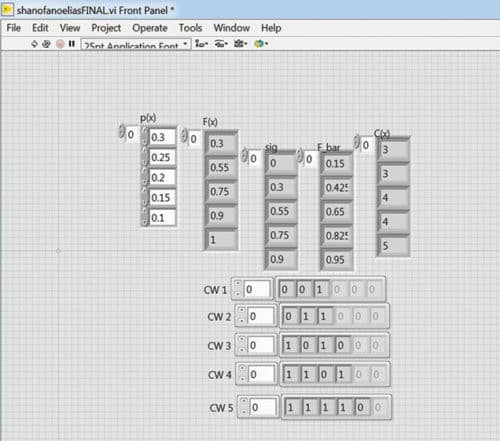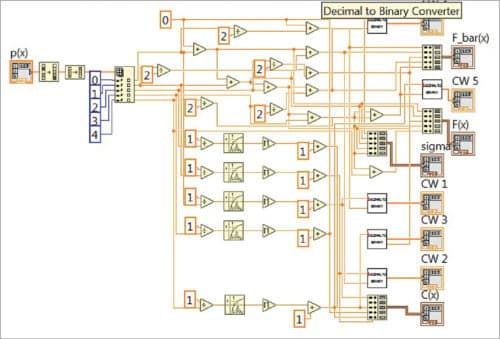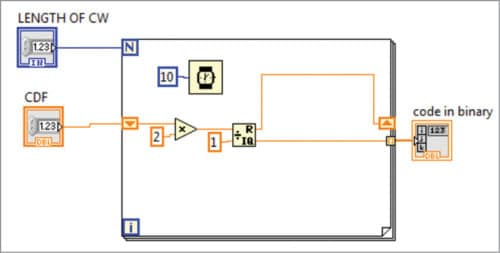Breaking News

# Implementation of Shannon Fano Elias Encoding Algorithm using LabVIEW

Shannon Fano Elias encoding algorithm is a precursor to arithmetic coding in which probabilities are used to determine code words. It is a lossless coding scheme used in digital communication. Probability theory has played an important role in electronics communication systems. It is because information in a signal is usually accompanied by noise. At the receiver end, when we talk about recovering the message signal, we are basically talking about estimating the original signal from the received signal accompanied by noise. In digital communication, we talk about decoding the receiving bits of a message with some probability of errors.

This article describes a simple construction procedure that uses cumulative distribution function (CDF) to generate code words using LabVIEW. The code word length of Shannon Fano Elias coding satisfies Kraft’s inequality. Therefore it is used to construct decodable code for the information source.

Shannon Fano Elias code procedure can be applied to a sequence of random variables. Probability and CDF can be calculated sequentially with symbols in a block or group of data, ensuring that the calculation grows linearly with block length. Direct application of Shannon Fano Elias code also needs arithmetic whose precision grows with block size.

## Encoding algorithm

In digital communication, the source encoder converts input, that is, symbol sequence, into a binary sequence of 0s and 1s. Each source symbol is represented by some sequence of coded symbols called code words.

Shannon Fano Elias encoding algorithm procedure is given as follows:

### Step 1

Arrange the symbols according to decreasing probabilities.

### Step 2

Calculate cumulative probability.

σ1=0; σ2=p1+σ1; σ3=p2+σ2; etc…

### Step 3

Calculate modified cumulative distribution function.

Fbar(x)=σi+p(xi)/2

### Step 4

Find the length of the code word.

l(x)=[log1/p(x)]+1

### Step 5

Generate the code word by finding the binary of Fbar(x) with respect to length l(x).

### Example

Consider a source with five symbols with probabilities 0.3, 0.25, 0.2, 0.15 and 0.1 (see table).

## Implementation of algorithm in LabVIEW

LabVIEW programs or virtual instruments (VIs) have front panels and block diagrams.

Front panel (Fig. 1) is the user interface. Block diagram (Fig. 2) is the programming behind the user interface. After building the front panel, the code is added in the block diagram using graphical representations of functions to control front panel objects. Code on the block diagram is graphical code, also known as G code or block diagram code.Fig. 1: Front panel of Shannon Fano Elias encodingFig. 2: Block diagram of Shannon Fano Elias encoding

Implementation of Shannon’s Fano Elias algorithm in LabVIEW requires the following components:

### Sort 1D array

This function returns a sorted version of the array with the elements arranged in ascending order. If the array is an array of clusters, the function sorts the elements by comparing the first elements. If the first elements match, the function compares the second and subsequent elements. The connector pane displays default data types for this polymorphic function.

### Reverse 1D array

This function reverses the order of elements in the array, where the array can be of any type. The connector pane displays default data types for this polymorphic function.

### Index array

This function returns the element or sub-array of n-dimension array at index. When you wire an array to this function, the function resizes automatically to display index inputs for each dimension in the array you wire to n-dimension array.

You can also add additional elements or sub-array terminals by resizing the function. The connector pane displays default data types for this polymorphic function.

### Divide

If you wire two waveform values or two dynamic data type values to this function, error in and error out terminals appear on the function. The connector pane displays default data types for this polymorphic function.

### Add

If you wire two waveform values or two dynamic data type values to this function, error in and error out terminals appear on the function. You cannot add two time-stamp values together. Dimensions of two matrices that you want to add must be the same.

Otherwise, this function returns an empty matrix. The connector pane displays default data types for this polymorphic function.

### Logarithm base 2

If x is 0, log2(x) is negative infinity. If x is not complex and is less than 0, log2(x) is NaN. The connector pane displays default data types for this polymorphic function.

### Round toward +infinity

For example, if input is 3.1, the result is 4. If input is -3.1, the result is -3. The connector pane displays default data types for this polymorphic function.

### Build cluster array

This function bundles each element input into a cluster and assembles all element clusters into an array of clusters. The connector pane displays default data types for this polymorphic function.

## Block diagram descriptionFig. 3: Decimal-to-binary conversion

### Step 1

Begin with the decimal fraction and multiply by 2. The whole number part of the result is the first binary digit to the right of the point.

This content was originally published here.

## Electronics Projects: Configurable RS232 To TTL To I2C Adaptor

RS232 signals cover a much longer distance than standard TTL and I2C signals. Also, RS232 …